# 一、JavaScript

1.创建正则表达式

var re = /ab+c/; //方式一 正则表达式字面量

var re = new RegExp("ab+c");  //方式二 RegExp对象的构造函

1）正则表达式字面量在脚本加载后编译。若你的正则表达式是常量，使用这种方式可以获得更好的性能。

2）使用构造函数，提供了对正则表达式运行时的编译。当你知道正则表达式的模式会发生改变， 或者你事先并不了解它的模式或者是从其他地方（比如用户的输入），得到的代码这时比较适合用构造函数的方式。

2.正则表达式中的特殊字符

\    ^    $* + ? . (x) (?:x) x(?=y) x(?!y) x|y {n} {n,m} [xyz] [^xyz] [\b] \b \B \cX \d \D \f \n \r \s \S \t \v \w \W \n \0 \xhh \uhhhh  3.正则表达式中的方法 6个，分别是exectestmatchsearchreplacesplit exec和test的语法都是regexObj调用，match、search、replace和split的语法都是string调用。 exec 方法为指定的一段字符串执行搜索匹配操作。它的返回值是一个数组或者 null。语法如下：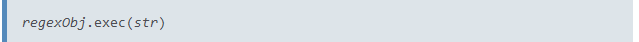示例代码： var re = /quick\s(brown).+?(jumps)/ig; var result = re.exec('The Quick Brown Fox Jumps Over The Lazy Dog'); 返回结果：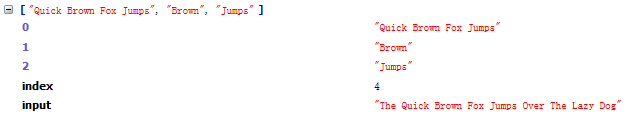test 一个在字符串中测试是否匹配的RegExp方法，它返回true或false。语法如下：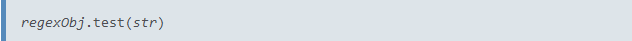match 一个在字符串中执行查找匹配的RegExp方法，它返回一个数组或者在未匹配到时返回null 与exec略有不同，首先是调用方式，math是由字符串调用，而exec是由RegexObj调用。 其次，如果表达式中有“g”标记，那么返回一个匹配的字符串数组，如果没有就会和exec返回的一样。下面这个demo就是有“g”。语法如下：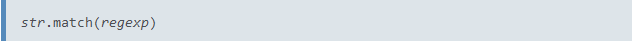示例代码： var re = /quick\s(brown).+?(jumps)/ig; var result = 'The Quick Brown Fox Jumps Over The Lazy Dog'.match(re); 返回结果：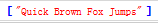search 一个在字符串中测试匹配的String方法，它返回匹配到的位置索引，或者在失败时返回-1。语法如下：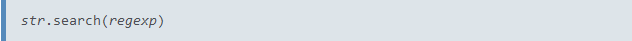例如上面的示例代码如果是调用search，返回数据就是4。 replace 一个在字符串中执行查找匹配的String方法，并且使用替换字符串替换掉匹配到的子字符串。语法如下：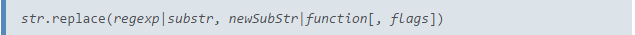var re = /(\w+)\s(\w+)/; var str = "John Smith"; var result = str.replace(re, "$2, $1"); 返回的结果将是：“Smith, John” split 一个使用正则表达式或者一个固定字符串分隔一个字符串，并将分隔后的子字符串存储到数组中的String方法。语法如下：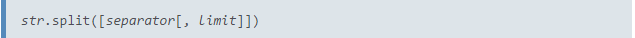limit就是限制分割后的数组中的个数。下面的是一个demo，但是表达式中有一个是加了括号，一个没加，返回的数据是不一样的。 示例代码： var re = /(\d)/; var result = 'Hello 1 word. Sentence number 2.'.split(re); console.log(result); var re = /\d/; var result = 'Hello 1 word. Sentence number 2.'.split(re); console.log(result); 返回结果：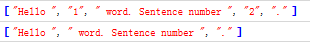4.正则表达式执行返回信息 var myRe = new RegExp("d(b+)d", "g"); var myArray = myRe.exec("cdbbdbsbz"); console.log(myArray); 代码中返回的结果如下：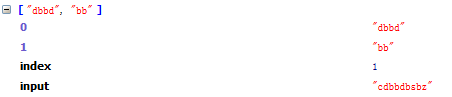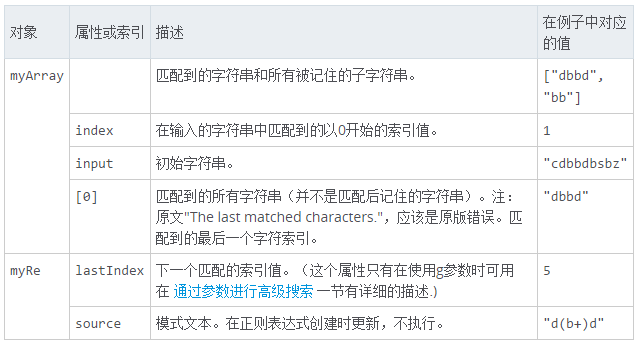5.正则表达式标志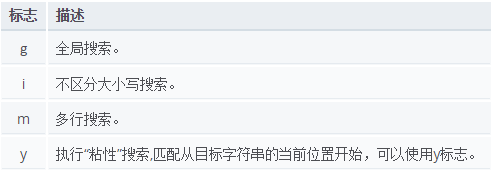var re = /\w+\s/g;//表达式一 var re = new RegExp("\\w+\\s", "g");//表达式二 var str = "fee fi fo fum"; var myArray = str.match(re); console.log(myArray); 表达式一和表达式二返回的结果是一样的。都是下面的那个数组：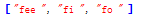# 二、PHP 下面的示例代码可以在ideone中查看到。 1.创建正则表达式 $regex = '/\d/i';

2.正则表达式中的特殊字符

$pattern = '/\d+/'; preg_match($pattern, $subject,$matches, PREG_OFFSET_CAPTURE);
print_r($matches); 上面的示例代码加了参数“PREG_OFFSET_CAPTURE”，这样的话，在$matches中会多一个偏移数。例如下面的“2”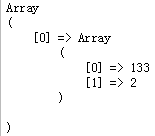preg_match_all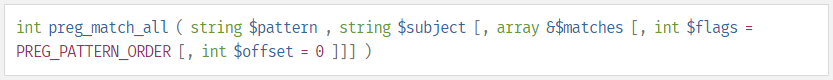preg_match_all($pattern,$subject, $matches, PREG_OFFSET_CAPTURE); print_r($matches);

$subject = array("dd133aa2", "kk1ff3");$pattern = '/\d+/';
$result = preg_replace($pattern, 'Z', $subject); print_r($result);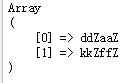preg_replace_callback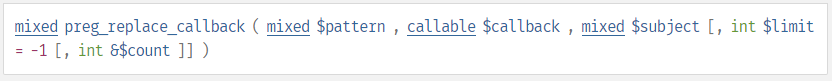$pattern = '/\d+/';$result = preg_replace_callback($pattern, function($matches) {
//$matches  => 133 //$matches  => 2
return 'Z';
}, $subject); print_r($result);

preg_grep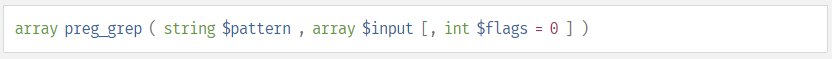$subject = array("dd133aa2", "kk1ff3", "ddsdfd");$pattern = '/\d+/';
$result = preg_grep($pattern, $subject);$result2 = preg_grep($pattern,$subject, PREG_GREP_INVERT);
print_r($result); print_r($result2);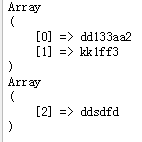preg_split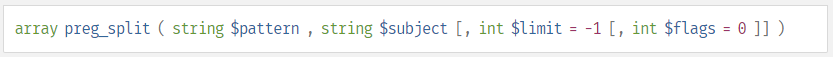$subject = "dd133aa2cc";$pattern = '/(\d+)/';
$result = preg_split($pattern, $subject);$result2 = preg_split($pattern,$subject, null, PREG_SPLIT_DELIM_CAPTURE);
print_r($result); print_r($result2);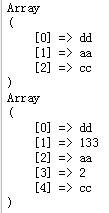preg_last_error

preg_match('/(?:\D+|<\d+>)*[!?]/', 'foobar foobar foobar');
$result = preg_last_error();//PREG_BACKTRACK_LIMIT_ERROR 调用回溯限制超出 print_r($result);

preg_quote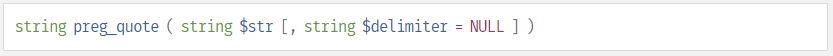$result = preg_quote($subject);
$result2 = preg_quote($subject, 'a');
print_r($result); print_r($result2);

4.模式修正符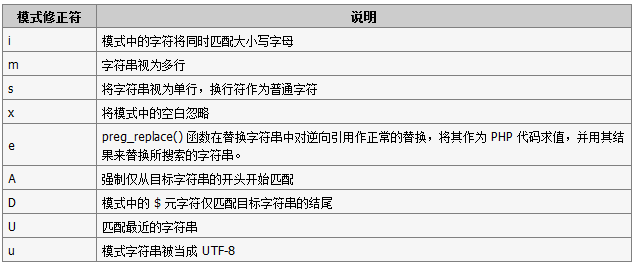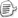posted on 2015-11-13 10:03  咖啡机（K.F.J）  阅读(1102)  评论(0编辑  收藏  举报